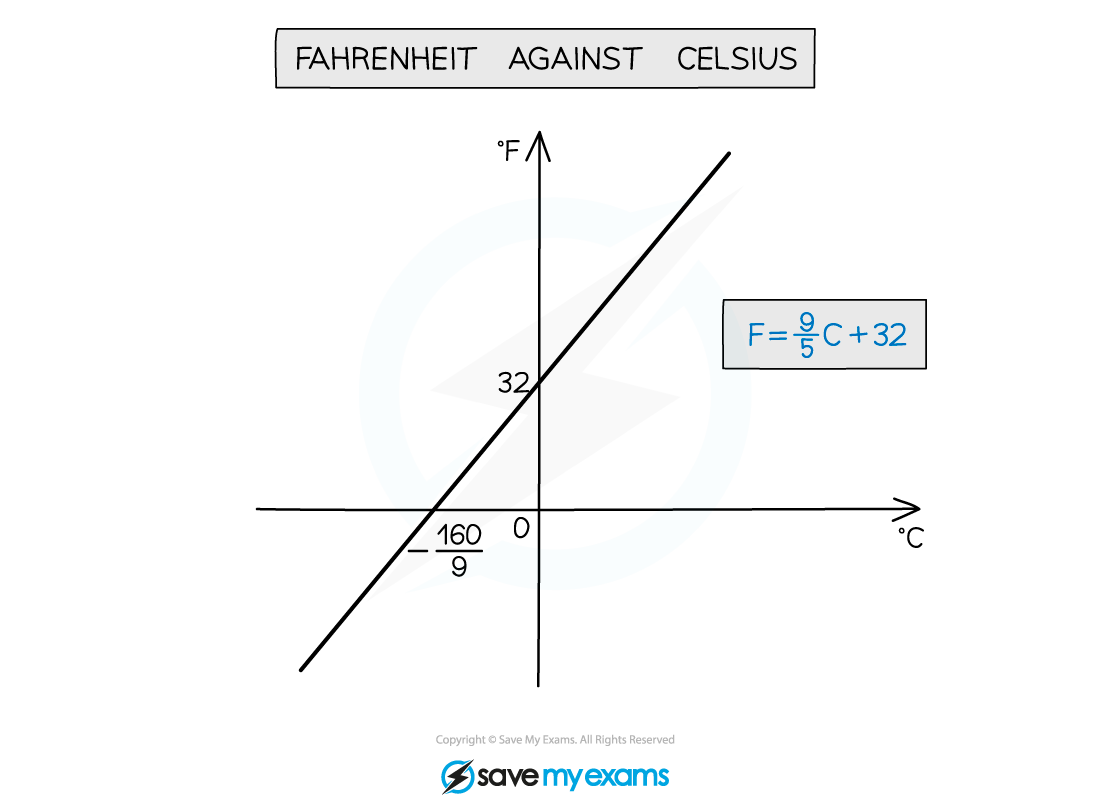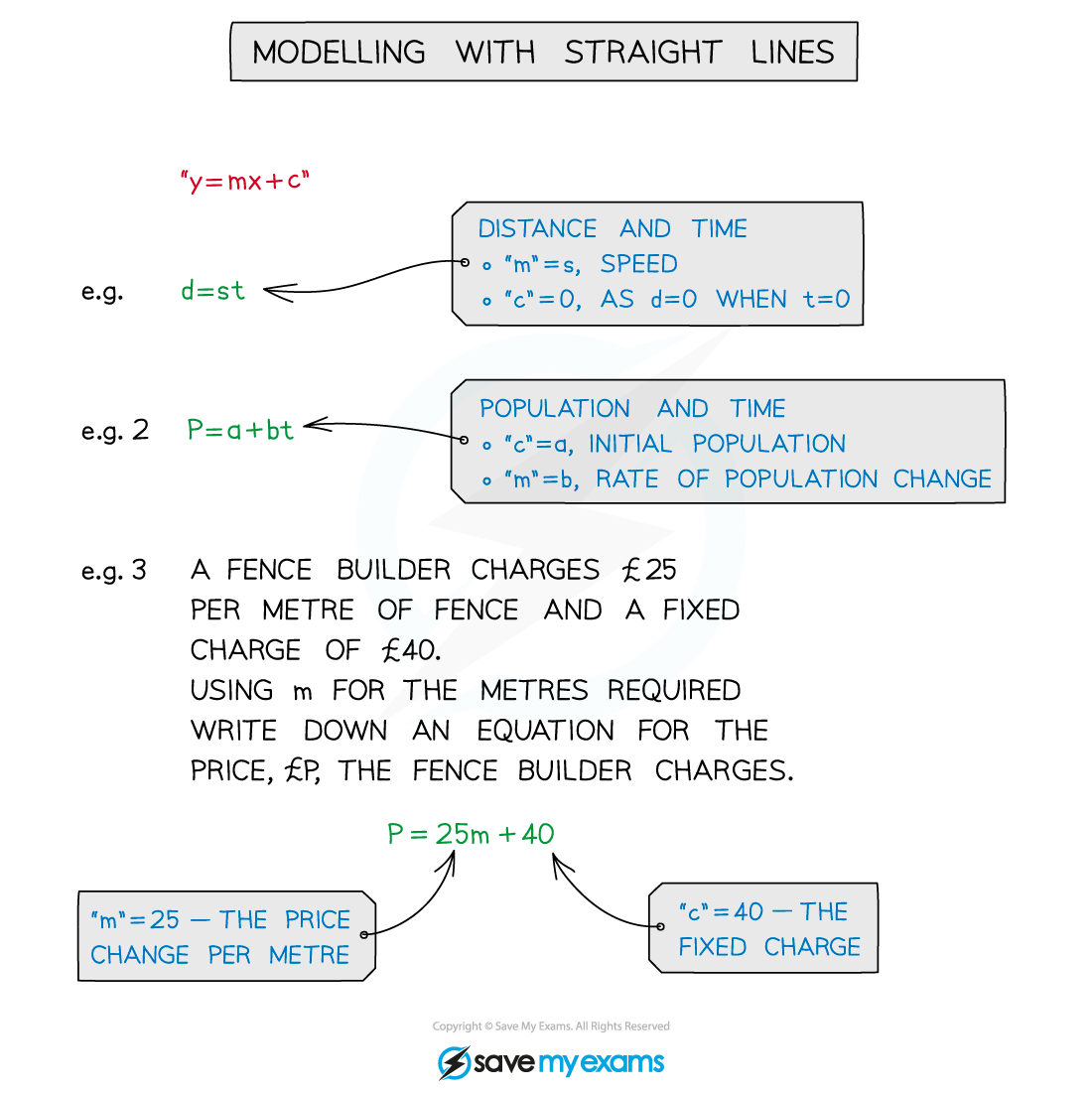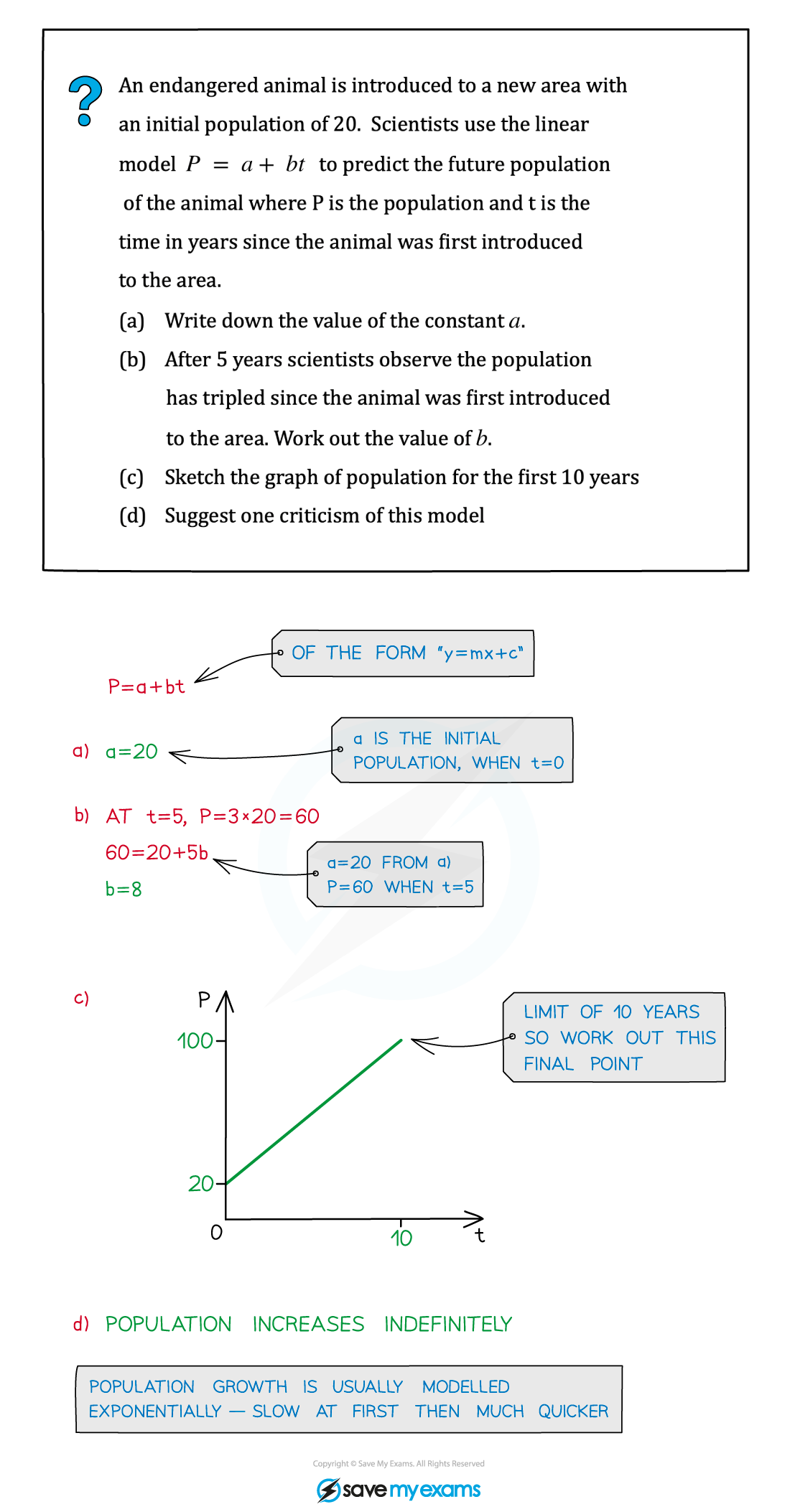# AQA A Level Maths: Pure复习笔记3.1.4 Modelling with Straight Lines

### Modelling with Straight Lines

#### What is modelling with straight lines?

• Modelling means applying the mathematics to a real-life situation
• The model is used to find or predict future values
• Models will often need refining to improve their accuracy#### How do I work with straight line models?

• Turn the words from the question into mathematical equations
• In y = mx + c constants “m” and “c” will have a meaning
• gradient “m” will be the rate of change
• eg extension in a spring per 1 kg of mass added
• y-axis intercept “c” will be the initial value
• eg resting length of a spring• The skills needed are covered in the other pages of the section Equation of a Straight Line

#### Worked Example